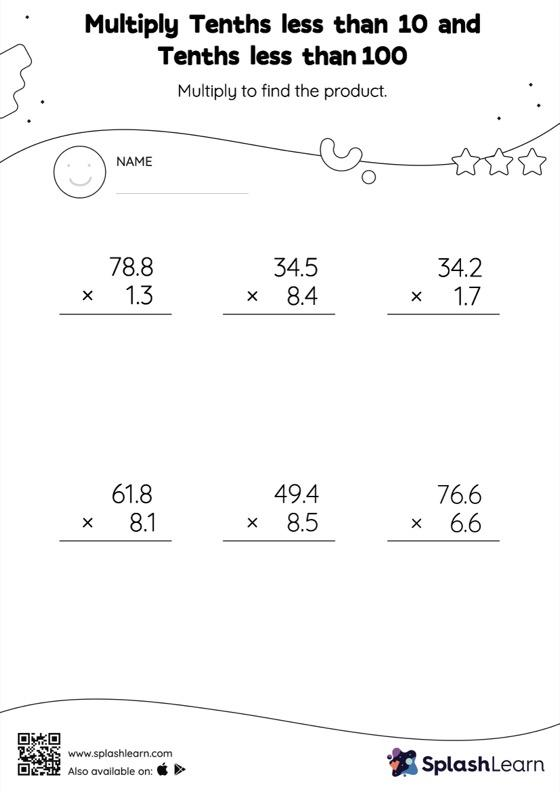# Multiply Tenths less than 10 and Tenths less than 100: Vertical Multiplication Worksheet

Home > Multiply Tenths less than 10 and Tenths less than 100: Vertical MultiplicationThis multiply tenths less than 10 and tenths less than 100 worksheet has a set of problems curated to ensure that your child becomes more proficient in multiplication. A tenth multiplied by another tenth will result in a product with two decimal digits. As they practice this idea in multiply tenths less than 10 and tenths less than 100 worksheet, they first multiply the numbers without accounting for the decimal points and then add the decimal point two places from the right in the product.